Browse by Topic
Related Topics

# New in the Wolfram Language: AnglePath

A brilliant aspect of the Wolfram Language is that not only you can do virtually anything with it, you can also do whatever you want in many different ways. You can choose the method you prefer, or even better, try several methods to understand your problem from different perspectives.

For example, when drawing a graphic, we usually specify the coordinates of its points or elements. But sometimes it’s simpler to express the graphic as a collection of relative displacements: move a distance r in a direction forming an angle θ with respect to the direction of the segment constructed in the previous step. This is known as turtle graphics in computer graphics, and is basically what the new function AnglePath does. If all steps have the same length, use AnglePath[{θ1,θ2,…}] to specify the angles. If each step has a different length, use AnglePath[{{r1,θ1},{r2,θ2}, …}] to give the pairs {length, angle}. That’s it. Let’s see some results.

Turn 60 degrees to the left with respect to the previous direction six times. You get a hexagon: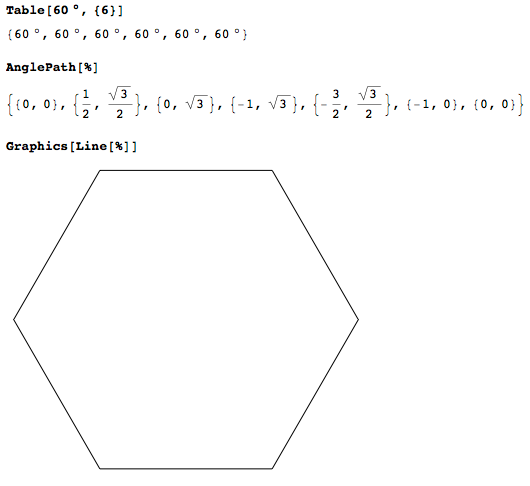If the angle is 135 degrees and you repeat eight times, then this is the result. Note that 8 * 135° = 1080°, so we go around the center three times: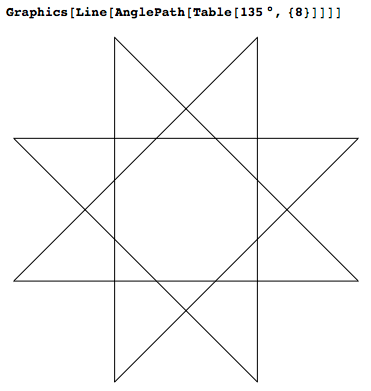Suppose that we again keep turning the same positive angle θ, but we increase the lengths of the steps linearly, in increments dr, from 0 to 1: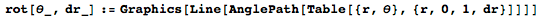Then we get these curves that spiral outward, producing nice outputs: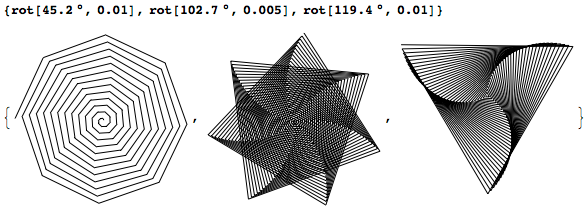If we choose the angles randomly, we get random walks on the plane: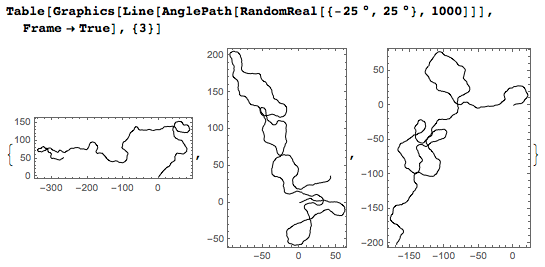Now let’s try to combine multiple AnglePath lines. Suppose that at each step we choose randomly between two possible angles and that the lengths obey a power law, so they get smaller in each iteration: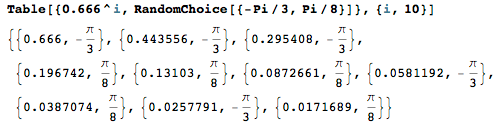The result does not look very interesting yet: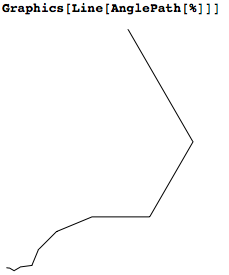But if we repeat the experiment 10 times, then we start seeing some structure: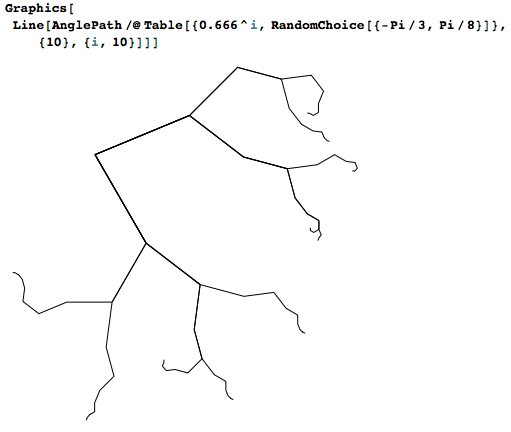We can construct all different lists of 10 choices of the two angles, using Tuples (there are 2^10=1024 possible lists). Replacing Line with BSplineCurve produces curved lines instead of straight segments. The result is a nice self-similar structure: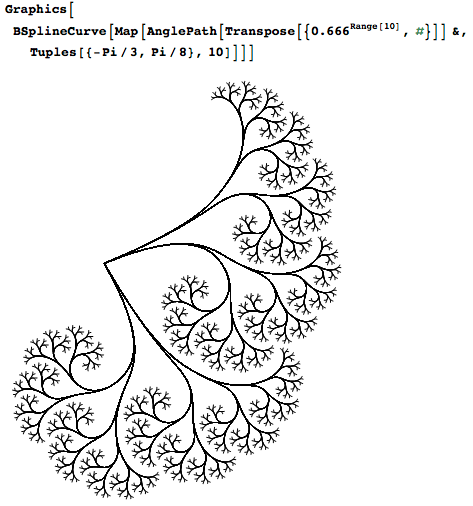AnglePath allows us to construct fractal structures easily, with very compact code. In fact, the code fits in a tweet! These are two examples derive from Wolfram Tweet-a-Program: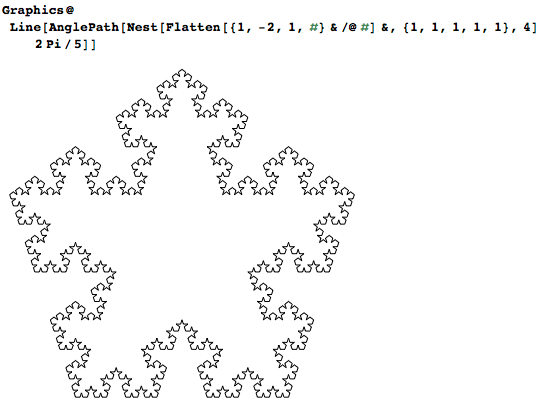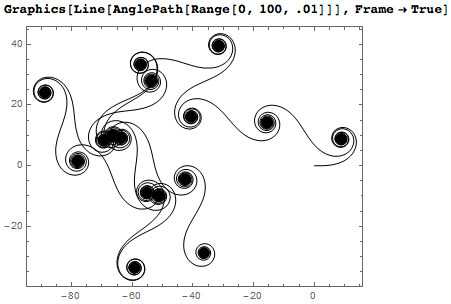These curious spirals are approximate Cornu spirals. With larger steps, they develop interesting substructure: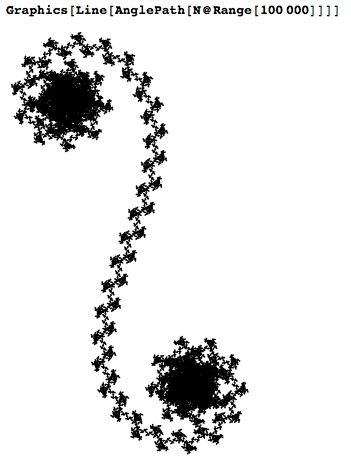This was a quick introduction to how useful and fun the function AnglePath can be. AnglePath is supported in Version 10.1 of the Wolfram Language and Mathematica, and is rolling out soon in all other Wolfram products. Start using it now, and tweet your results through Wolfram TaP!